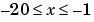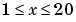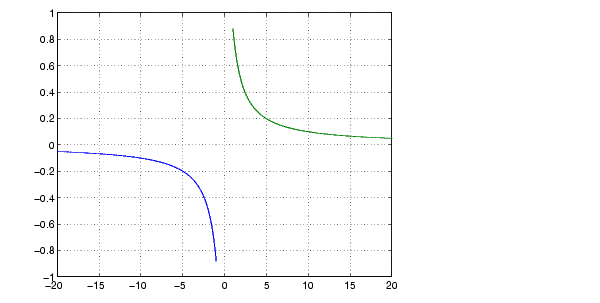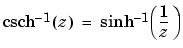MATLAB Function Referenceacsch

Inverse cosecant and inverse hyperbolic cosecant

Syntax

• ```Y = acsch(X)
```

Description

```Y = acsch(X) ``` returns the inverse hyperbolic cosecant for each element of `X`.

The `acsch` function operates element-wise on arrays. The function's domains and ranges include complex values. All angles are in radians.

Examples

Graph the inverse hyperbolic cosecant over the domainsand.

• ```x1 = -20:0.01:-1;
x2 = 1:0.01:20;
plot(x1,acsch(x1),x2,acsch(x2)), grid on```

Definition

The hyperbolic inverse cosecant can be defined as

•Algorithm

`acsc` uses FDLIBM, which was developed at SunSoft, a Sun Microsystems, Inc. business, by Kwok C. Ng, and others. For information about FDLIBM, see http://www.netlib.org.

See Also

`acsc`, `csch`

© 1994-2005 The MathWorks, Inc.